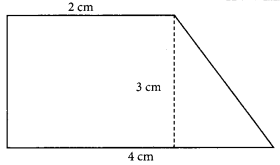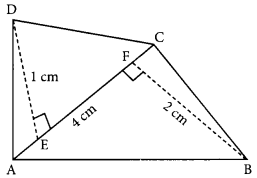# MCQ Questions for Class 8 Maths Chapter 11 Mensuration with Answers

We have compiled the NCERT MCQ Questions for Class 8 Maths Chapter 11 Mensuration with Answers Pdf free download covering the entire syllabus. Practice MCQ Questions for Class 8 Maths with Answers on a daily basis and score well in exams. Refer to the Mensuration Class 8 MCQs Questions with Answers here along with a detailed explanation.

## Mensuration Class 8 MCQs Questions with Answers

Choose the correct option.

Question 1.
Volume of cuboid having length 5.1 m, breadth 2 m and height 2 m.
(a) 19.4 m²
(b) 20.4 m²
(c) 21.2 m²
(d) 24.4 m²

Question 2.
The area of a rhombus is 70 cm². One diagonal is 14 cm. The other diagonal is
(a) 20 cm
(b) 10 cm
(c) 5 cm
(d) 15 cm

Question 3.
The area of the trapezium is(a) 9 cm²
(b) 6 cm²
(c) 7 cm²
(d) 24 cm²

Question 4.
The area of a rhombus is 60 cm² and one diagonal is 10 cm. The other diagonal is
(a) 6 cm
(b) 12 cm
(c) 3 cm
(d) 24 cm

Question 5.
The area of the quadrilateral is(a) 8 cm²
(b) 12 cm²
(c) 6 cm²
(d) 3 cm²

Question 6.
The height of two right circular cylinders are the same. Their volume’s are respectively 16 π m³ and 81 π m³. The ratio of their base radius is
(a) 16 : 81
(b) 9 : 14
(c) 2 : 3
(d) 4 : 9

Question 7.
The ratio of the radius of two right circular cylinder is 1 : 2 and the ratio of their height is 4 : 1 then the ratio of their volume is
(a) 1 : 2
(b) 2 : 1
(c) 1 : 1
(d) 4: 1

Question 8.
If base area of a room is 30 m² and height is 3 m then its volume is ………..
(a) 900 m³
(b) 90 m³
(c) 33 m³
(d) 33 m³

Question 9.
Two identical cubes each of total surface area 24 cm² are joined end to end. Which of the following is the total surface area of the cuboid so formed?
(a) 144 cm²
(b) 40 cm²
(c) 120 cm²
(d) 118 cm²

Question 10.
The base area of a right circular cylinder is 16 π cm³. Its height is 5 cm. Its curved surface area is …………….
(a) 40 π cm²
(b) 30 π cm²
(c) 20 π cm²
(d) 10 π cm²

Question 11.
Which of the following is the once of a rhombus?
(a) Product of its diagonals
(b) $$\frac {1}{2}$$ (sum of its diagonals)
(c) $$\frac {1}{2}$$ (Product of its diagonals)
(d) 2 (Product of its diagonals)

Answer: (c) $$\frac {1}{2}$$ (Product of its diagonals)

Question 12.
If the edge of a cube is 1cm then which of the following is its volume?
(a) 6 m³
(b) 3 m³
(c) 1 m³
(d) none of these

Fill in the blanks

Question 1.
If the side of a cube is doubled, the volume is ………………….. times greater.

Question 2.
The edge of a cube is 24 cm, ………………….. cubes of side 8 cm be formed from this cube.

Question 3.
The radius of a cylinder with lateral surface area 440 cm² and height 14 cm is …………………..

Question 4.
The ratio of the radii of two circular cylinder of equal volume and heights in the ratio 2 : 1 is …………………..